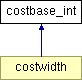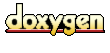Main Page   Class Hierarchy   Compound List   File List   Compound Members   File Members

# costbase_int Class Reference

Base class for objects that define integer costs functions for an integer version of the wavelet packet transform. More...

`#include <costbase_int.h>`

Inheritance diagram for costbase_int::List of all members.

## Public Methods

costbase_int ()
The default constructor does nothing. More...

## Protected Methods

void traverse (packnode< int > *node)
Recursively traverse the wavelet packet tree and calculate the cost function. More...

virtual int costCalc (packnode< int > *node)=0
Cost function to be defined by the subclass. More...

## Private Methods

costbase_int (const costbase_int &rhs)
disallow the copy constructor. More...

## Detailed Description

Base class for objects that define integer costs functions for an integer version of the wavelet packet transform.

The costbase base class provides a constructor that is passed the root of an integer wavelet packet tree (which is built by packtree_int.cpp). The integer wavelet packet tree is constructed from packnode<int> objects (which are a subclass of the packdata).

The cost function calculation invoked by the constructor traverses the wavelet packet tree (top down) and calls costCalc on each node in the tree. The cost function result is stored in the node. Note that the cost function also calculates a cost value for the original data, since in theory the original data may represent the minimal representation for the data in terms of the cost function.

The pure virtual function costCalc, which calculates the cost function, must be defined by the subclass.

A description of the cost functions associated with the wavelet packet transform can be found in Chapter 8 of Ripples in Mathematics by Jense and la Cour-Harbo.

Definition at line 66 of file costbase_int.h.

## Constructor & Destructor Documentation

 costbase_int::costbase_int ( const costbase_int & rhs )` [inline, private]`
 disallow the copy constructor. Definition at line 70 of file costbase_int.h.```00070 {} ```

 costbase_int::costbase_int ( )` [inline]`
 The default constructor does nothing. Definition at line 93 of file costbase_int.h.```00093 {} ```

## Member Function Documentation

 int costbase_int::costCalc ( packnode< int > * root )` [protected, pure virtual]`
 Cost function to be defined by the subclass. Reimplemented in costwidth. Referenced by traverse().

 void costbase_int::traverse ( packnode< int > * node )` [inline, protected]`
 Recursively traverse the wavelet packet tree and calculate the cost function. Definition at line 77 of file costbase_int.h. Referenced by costwidth::costwidth(). ```00078 { 00079 if (node != 0) { 00080 int cost = costCalc( node ); 00081 node->cost( cost ); 00082 00083 traverse( node->lhsChild() ); 00084 traverse( node->rhsChild() ); 00085 } 00086 } // traverse ```

The documentation for this class was generated from the following file:
Generated at Sat Aug 10 13:23:36 2002 for Wavelet Packet Transform and Lossless Compression by1.2.8.1 written by Dimitri van Heesch, © 1997-2001